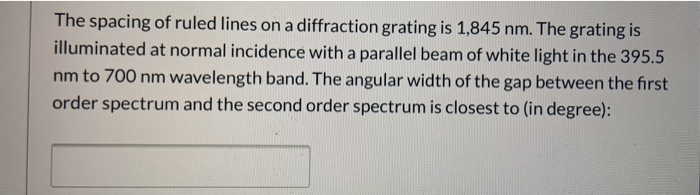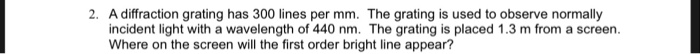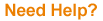Question

A diffraction grating with 230 lines per mm is used in an experiment to study the visible spectrum of a gas discharge tube. At what angle from the beam axis will the first order peak occur if the tube emits light with wavelength of 421.2 nm?

At what angle will the second order peak occur?

Solution)

We know,

d sin theta= wavelength

d = 1/230 per mm = 0.0043 mm X (10^6nm/mm) = 4347.82 nm

theta = first peak = arc sin (wavelength/d)

= 5.55 degrees

Now,

second peak = arc sin (2 *wavelength/d)

= 11.17 degrees

===========

#### Earn Coins

Coins can be redeemed for fabulous gifts.

Similar Homework Help Questions
• ### ANGLE OF FIRST AND SECOND ORDER MAXIMA IN DIFFRACTION PATTERN!

A diffraction grating with 280 lines per mm is used in an experiment to study the visible spectrum of a gas discharge tube.1)At what angle from the beam axis will the first order peak occur if the tube emits light with wavelength of 585.5 nm?2)At what angle will the second order peak occur?

• ### Diffraction grating

A diffraction grating with 13400 lines per 2.54 cm (i.e., per inch) is used to view the visible spectrum from hydrogen gas excited by an electrical discharge. At whatangle from the beam axis will the first order peak for the red photons (wavelength 656 nm) occur?Please show all the work, it is much appreciated!

• ### Intense white light is incident on a diffraction grating that has 460 lines/mm. (a) What is...

Intense white light is incident on a diffraction grating that has 460 lines/mm. (a) What is the highest order in which the complete visible spectrum can be seen with this grating? (Enter 1 for first order, 2 for second order, etc.) (b) What is the angular separation between the violet edge (400 nm) and the red edge (700 nm) of the first order spectrum produced by the grating?

• ### A 500 lines per mm diffraction grating is illuminated by light of wavelength 560 nm ....

A 500 lines per mm diffraction grating is illuminated by light of wavelength 560 nm . Part A What is the maximum diffraction order seen Part B: What is the angle of each diffraction order starting from zero diffraction order to the maximum visible diffraction order? Enter your answers in degrees in ascending order separated by commas.

• ### ASAP PLEASE The spacing of ruled lines on a diffraction grating is 1,845 nm. The grating...ASAP PLEASE The spacing of ruled lines on a diffraction grating is 1,845 nm. The grating is illuminated at normal incidence with a parallel beam of white light in the 395.5 nm to 700 nm wavelength band. The angular width of the gap between the first order spectrum and the second order spectrum is closest to (in degree):

• ### A 500 lines per mm diffraction grating is illuminated by light of wavelength 640 nm .

A 500 lines per mm diffraction grating is illuminated by light of wavelength 640 nm . For the steps and strategies involved in solving a similar problem, you may view a Video Tutor Solution.Part AWhat is the maximum diffraction order seen? Express your answer as an integer. Part B What is the angle of each diffraction order starting from zero diffraction order to the maximum visible diffraction order?

• ### A 500 lines per mm diffraction grating is illuminated by light of wavelength 510...continues

A 500 lines per mm diffraction grating is illuminated by light of wavelength 510 nm. What is the angle of each diffraction order?

• ### 2. A diffraction grating has 300 lines per mm. The grating is used to observe normally...2. A diffraction grating has 300 lines per mm. The grating is used to observe normally incident light with a wavelength of 440 nm. The grating is placed 1.3 m from a screen. Where on the screen will the first order bright line appear?

• ### White light is incident on a diffraction grating with 479 lines/mm. HINT (a) Calculate the angle...White light is incident on a diffraction grating with 479 lines/mm. HINT (a) Calculate the angle θr2 (in degrees) to the second-order maximum for a wavelength of 620 nm. ° (b) Calculate the wavelength in nm of light with a third-order maximum at the same angle θr2. nm

• ### If the scientist uses a diffraction grating with 500 lines per mm and laser with a...

If the scientist uses a diffraction grating with 500 lines per mm and laser with a wavelength of 680 nm, what angle will a line from the grating to the second order bright spot make with the line from the grating to the central bright spot?